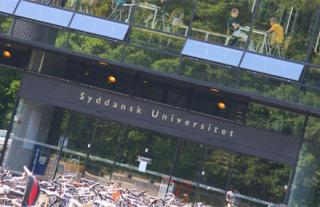# Mathematic methods in Chemistry and Nanoscience (KE529: 5 ECTS)The purpose of this course is to introduce mathematical notation and mathematical methods for analysis of chemical problems. Emphasis will be on practical / computing aspects of the mathematical methods introduced in the course. The course also provides an introduction to the use of Maple for the analysis of more mathematically complex chemical problems.

Topics such as 1st and 2nd order differential equations and basic linear algebra (matrices, eigenvalues, eigenvectors, diagonalization) is covered.

You can read more in the course description.

Date
01-09-2016
26-11-2016
Institution
University of Southern Denmark, Department of Physics Chemistry and Pharmacy
Role
Teaching assistant
Teacher
Claus Nielsen